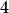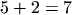## A.4.5 Local Modifications in Inherited Sections

Inherited sections may be locally modified. This can be done by specifying a section body, e.g.,

```Section24
{  x = 1;
y = 2;
}

Section25 : Section24
{  y = 4;
}
```

The section Section25 consists of the two variables x and y, where the variable x is inherited from Section24 and the variable y is locally modified. So the variable `~`Section25.x equalsand the variable `~`Section25.y equals.

Inheritance of variables is a powerful feature. See the example below:

```Section26
{  x = 1;
y = x + 2;
}

Section27 : Section26;
```

The section Section27 now consists of two variables (x and y) which are inherited from Section26. The variable y in section Section27 was inherited form Section26, where the variable y is referring to a variable named x. Changes made to variable x within section Section26 (the parent section) will cause the values of the variables y in both sections (Section26 and Section27) to change.

An important different behavior can be observed if the value of variable x within section Section27 is locally modified, e.g.,

```Section27 : Section26
{  x = 5;
}
```

The section Section27 again consists of two variables (x and y), where variable x is locally modified and variable y is inherited from Section26.

The variable y within section Section27 was inherited from section Section26, where the variable y is referencing a variable named x. Since variable x has been locally modified within section Section27, inquiring the value of variable y of section Section27 will deliver the value.

When using inherited sections, only those elements can be locally modified that are passed on to this section.

For instance, the following modification of section Section27 will be illegal:

```Section27 : Section26
{  z = 9;                       // Error! `z' is not an element of Section26.
}
```

In this case the an error is created. In other words, by default an inherited section is protected from appending new entries. This allows to detect misspelled variable and section names in inherited sections and prevents from accidentally creating new ones. If the Input Deck database would not act like this a new variable (z) would be appended.

This behavior can be explicitly disabled by enclosing the section-name in angle brackets, e.g.,

```<Section27> : Section26
{  y = 7;
z = 9;                       // no error
}
```

Now section Section27 consists of the three variables (x, y, and z), where the variable x is inherited from Section26, variable y is locally modified, and the variable z is appended.

However, a better solution to add new variables is to use the plus (+) sign preceding the variable name.

```X
{  a = yes;
b = 3;
}

Y : X // inherit Y from X
{  c = "Test";                  // can not be added, ERROR
+d = "Test";                  // OK
}
```

Section Y now contains a = yes, b = 3, and d = "Test".

Robert Klima 2003-02-06